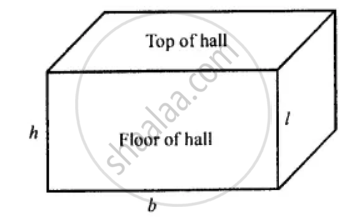# The Floor of a Rectangular Hall Has a Perimeter 250 M. If the Cost of Painting the Four Walls at the Rate of ₹ 10 per M2 is ₹ 15,000, Find the Height of the Hall. - Mathematics

Sum

The floor of a rectangular hall has a perimeter 250 m. If the cost of painting the four walls at the rate of ₹ 10 per m2 is ₹ 15,000, find the height of the hall.

#### Solution

Let the length, breadth, and height of the rectangular hall be l m, b m, and h m respectively.The perimeter of the floor of hall = 2(l + b)

250 m = 2(l + b)

(l + b) = 250/2 = 125 cm   ...(i)

Area of four walls = Area of cuboid – Area of floor – Area of top

= 2 (lb + bh + hl) – (l x b) – (l x b)

= 2(lb) + 2 (bh) + 2(hl) – 2lb = 2 lh + 2 bh

= 2h(l + b)

= 2h x 125 [From (i)]

= 250h m2

Area of four walls = 250h m2

Cost of painting 1 m2 area = ₹ 10

Cost of painting 250h m2 area = ₹ 10 x 250h = 2500h
15000 = 2500h

h = 15000/2500

The height of the hall is 6 m.

Concept: Surface Area of a Cuboid
Is there an error in this question or solution?

#### APPEARS IN

Selina Concise Mathematics Class 8 ICSE
Chapter 21 Surface Area, Volume and Capacity
Exercise 21 (E) | Q 6 | Page 244